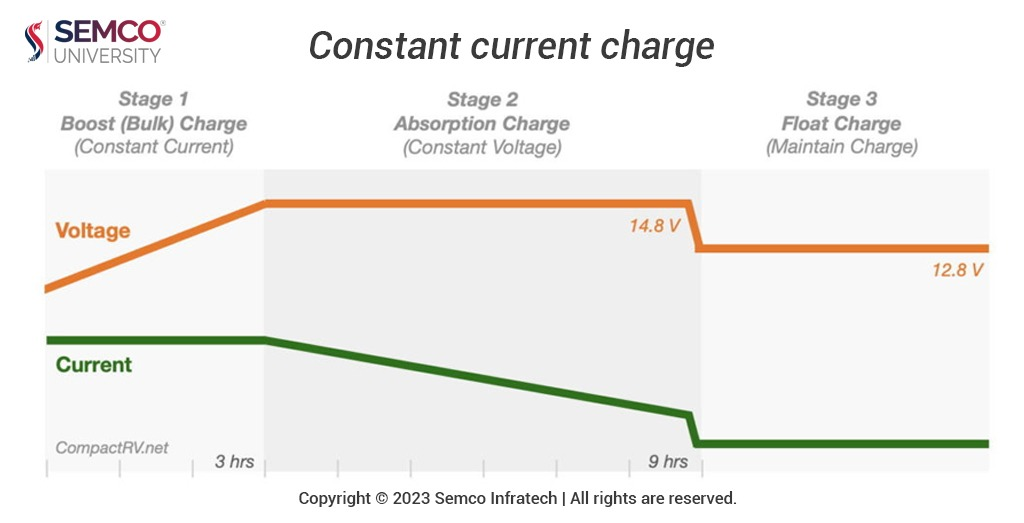Semco university – All about the Lithium-Ion Batteries# Understanding CITT Testing and Lithium-ion Diffusion ModelsIntroduction

In the realm of lithium batteries, precise testing methods and theoretical models play a vital role in understanding their performance characteristics. This article delves into the CITT (Constant Current-Constant Voltage) testing method and explores the theoretical model of lithium-ion diffusion, shedding light on how these techniques provide valuable insights into battery behavior.

CITT Testing Method

The CITT testing method is a crucial tool in evaluating lithium battery performance. It involves a series of steps that allow for a comprehensive assessment of a battery’s behavior under various charging and discharging conditions:

Initial Charge/Discharge: The battery is subjected to a preliminary charge/discharge cycle at a rate of 0.2 C5A (where C5 is a 5-hour rate discharge capacity). The voltage parameters for this step include a discharge cut-off voltage of 2.75V and a charging cut-off voltage of 4.2V. After this cycle, the open circuit voltage (UOCV) is measured following a 1-hour rest period.

Constant Current Charging: The CITT capacity method test begins with constant current (CC) charging to a voltage of 3.5V. This initial charging phase is followed by constant voltage (CV) charging until the current approaches zero (0.01 C5A). This phase aims to stabilize the electrochemical environment within the battery.

Repeated Charging: The CC-CV charging process is repeated, gradually increasing the voltage to reach 4.2V. The voltage intervals for CC-CV charging can be adjusted as needed. After each CITT capacity test, the battery is discharged to 2.75V at a rate of 0.2 C5A, and the next cycle of CITT testing commences.

The CITT testing method allows researchers and manufacturers to observe how a battery performs under controlled conditions, providing valuable data on its capacity, voltage behavior, and stability.

Theoretical Model of Lithium Ion Diffusion

Understanding the diffusion of lithium ions within a battery is essential for predicting its behavior accurately. One theoretical model used to describe this diffusion is the spherical diffusion model. This model relates the constant voltage-constant current charging capacity ratio (q) to various parameters:

ξ (dimensionless): A constant representing the lithium-ion concentration boundary condition.

R (particle radius, cm): The radius of the solid particles within the battery.

tG (constant current charging time, s): The duration of the CC charging phase.

D (solid phase diffusion coefficient, cm²/s): A key parameter describing the rate of lithium-ion diffusion.

The formulae for q and ξ can be expressed as per the following equations:

q = ξ(1 – (1 – (a/R)²) * exp(-a²DtG/R²)) …(Formula 1)

ξ = (1 – (1 – (a/R)²) * exp(-a²DtG/R²)) / (1 – (1 – (a/R)²) * exp(-2a²D*tG/R²)) …(Formula 2)

In these equations, a represents a constant sequence, and its values for the first nine roots are given as per the table below:

Data Analysis

To determine the diffusion coefficient D, series equations of D=f(q) are linearly fitted using the least squares method for different q values. This process enables the establishment of a series of equations that describe D in terms of q. By measuring the particle radius (R), CC-CV charging capacity ratio (q), and constant current charging time (tG), researchers can obtain the diffusion coefficient D.

Understanding Lithium Ion Diffusion

Lithium ion diffusion within battery materials is a complex phenomenon, but theoretical models like the spherical diffusion model offer a systematic framework for analysis. These models help researchers gain insights into how lithium ions move within solid materials, contributing to the development of better-performing lithium batteries.

Conclusion

The CITT testing method and theoretical models of lithium ion diffusion are indispensable tools in the realm of battery research and development. These techniques allow for a deeper understanding of battery behavior, aiding in the quest to create more efficient, reliable, and safe lithium battery technologies.

About Semco – Semco University is an educational website that is catering to the needs of students and researchers. Offering information on Lithium-ion batteries. The resources and content are compiled from various sources including manufacturers, test labs, crowdsourcing, etc. Our motto is to provide a viable resource for companies, students, and enthusiasts interested in participating in the Li-ion Battery industry. Our initiative is to make people aware of the benefits, and opportunities of the revolutionary Lithium Batteries for multiple applications.